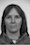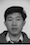## Part 1A: Run PCA on Iris Dataset and Visualize First Two Z-Scores

Run the principal component analysis on Fisher' Iris dataset (using this version). Insert the mean vector, the eigenvalues, and the corresponding eigenvectors into the table below. Also, visualize the dataset in 2D using a scatter plot of the first principal component score versus the second principal component score. Use different markers to indicate the three different classes of instances in the dataset. Preferably, your plot should appear in full color. Also, provide a CSV or Excel file with the component scores that were used to generate the scatter plot.

Fill the missing values in the mean vector:

 Sepal length: Sepal width: Petal length: Petal width: ? 3.06 ? ?

Complete the following table with the eigenvalues and the corresponding eigenvectors of the covariance matrix, starting from the largest eigenvalue.

 1 2 3 4 Egenvalue: ? 0.24 ? 0.02 Sepal length: ? ? ? ? Sepal width: ? ? ? ? Petal length: ? ? ? ? Petal width: ? ? ? ?

 Example scatter plot in grayscale:Insert your scatter plot here:## Part 1B: Visualization Inspired by Eigenfaces

Use PCA on the space of pixels in grayscale to visualize several low-resolution pictures of faces in a 2D grid where the two axes correspond to the scores for the first two principal components (the required computation is similar to what the Eigenfaces algorithm does). Use any program that can position graphics over other graphics, e.g., Microsoft PowerPoint, to arrange images on the scatterplot to indicate which points correspond to which images. First, update the course app in the VM to version 0.9.8 or later using the 'Update App' desktop shortcut. Second, convert the image to CSV using the 'Images to CSV' tool within the app. Finally, use the resulting CSV files to perform PCA and the visualiztion. The input data is given in the following table:An example greyscale figure that should look similar to your results is shown below.Insert your plot to replace the placeholder above.

## Part 1C: Extend Visualization to Images Outside Training Set

Extend the visualization developed in Part 1B to the two new images that weren't used for training without re-running PCA, i.e., by re-using the mean vector and the eigenvectors from Part 1B. The two additional faces are shown in the following table:An example greyscale figure that should look similar to your results is shown below.Insert your plot to replace the placeholder above.

## Part 1D: Analyze PCA Residuals for Images Used in Part 1C

For each of the 11 images used in Part 1C, compute M, which is equal to the magnitude of the centered observation vector, i.e., the vector obtained by subtracting the mean vector from the vector formed by the image pixels. Also, compute R, i.e., the magnitude of the residual that is not explained by the first two principal components. This can be done by computing the projections of the centered observation vector on the first and second principal component, subtracting them from the centered observation vector and computing the magnitude of the resulting vector. Use the results to compute P, i.e., the percentage of the image explained by the first two principal components, i.e., P = 100*(1 - R/M).

Finally, perform the same analysis for the white noise image (see below). Use the results to complete the following table.

 Image M R P2230.116 1921.019 13.8601781.570 ? 45.8221219.905 ? ?? ? 67.084? ? ?? ? ?? ? ?? ? ?? ? 35.9512528.428 ? ?? ? ?? 3319.578 ?

## Part 2A: Train One-vs-Rest Linear SVM on Two PC Scores

Update the course app in the VM to version 0.9.9.1 or later using the 'Update App' desktop shortcut. Then, use the linear SVM tool in the app to determine the equations of three lines that separate each of the three classes in the Iris dataset from the remaining two classes in the space of the first two principal component scores generated in Part 1A. One line should clearly split the leftmost cluster. Another line should clearly split the rightmost cluster. The third line that corresponds to the middle cluster should pass roughly horizontally through the dataset because the middle cluster cannot be easily separated from the other two by only one line.

Use the coefficients for the three separating lines to replace the question marks below:

 Equation for Iris setosa: ? x PC1 + ? x PC2 + ? = 0 Equation for Iris versicolor: ? x PC1 + ? x PC2 + ? = 0 Equation for Iris virginica: ? x PC1 + ? x PC2 + ? = 0

## Part 2B: Train Linear SVM on Wine Dataset

Update the course app in the VM to version 0.9.9.1 or later using the 'Update App' desktop shortcut. Then, train a linear SVM on the wine dataset and state the equation for the hyperplane that separates the two classes. Use the following two files for training: wine_features.csv and wine_labels.csv (they can be downloaded in the VM by updating the datasets). Hint: the weights for the features are stored as the list coef in the linear SVM model CSV file. The value of w0 is called the intercept.

Use the coefficients for the separating hyperplane to replace the question marks below:

 ? x fixed acidity + ? x volatile acidity + ? x citric acid + ? x residual sugar + ? x chlorides + ? x free sulfur dioxide + ? x total sulfur dioxide + ? x density + ? x pH + ? x sulphates + ? x alcohol + ? = 0

## Part 2C: Implement Linear SVM Decision Function in Excel

Use the hyperplane coefficients from Part 2B to implement the linear SVM classification in Excel. Then, measure its accuracy on the training set, i.e., compare the labels predicted for the instances in wine_features.csv with the ground truth in wine_labels.csv and find the percentage of correctly predicted labels (it should be close to 98% or higher).

Fill the missing values in the mean vector:

 Accuracy: ? Number of errors: ? Number of correctly predicted labels: ?

## [HCI Implementation, Optional] Part 3: Patch the Gaussian Mixtures Tool in the Course App to Sort Eigenvalues in Decreasing Order

Locate the python source code of the course app in the VM and hack it so that it reports eigenvalues and eigenvectors of the covariance matrices for Gaussian mixture components starting from the largest eigenvalue in decreasing order instead of the default behavior in version 0.9.11.2 when they are sorted in increasing order. This problem should be solvable even if you don't know Python. The source code can be found by exploring the VM. Insert your patch in the space below.

--- lao	2002-02-21 23:30:39.942229878 -0800
+++ tzu	2002-02-21 23:30:50.442260588 -0800
@@ -1,7 +1,6 @@
-Replace this example patch
+With your patch for the course app that sorts eigenvalues better.


## Part 4 (Extra Credit): Derive a Basis Function for a Given Kernel Function

Find the basis function $\varphi(x)$ for the kernel $K(x,y) = (x \, y + c)^2$, where x and y are 1-dimensional feature vectors and c is a parameter. Hint: this kernel function is a special case of the polynomial kernel.

Insert your basis function here: $\varphi(x) = \quad ?$